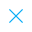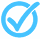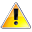Call Support +91-85588-96644

Keep me logged in
You can't leave Captcha Code empty
By submitting this form, you agree to the Terms & Privacy Policy.
ORTests given# Linear Equations in Two Variables - 2 By TCY All tests

Questions

Time

Highest
score

Level

## ENGLISH

Language

Topics Covered:Linear Equations with One and Two VariablesLinear EquationsSolutions of Linear Equations in 2 VariablesSolving a Pair of Linear EquationsPlotting Solutions of Linear EquationsSolution of a Linear Equation in 2 VariablesLinear Equations in 2 VariablesLinear Equations in Two VariablesPair of Linear Equationsin 2 Variables